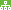How to Learn in 24 Hours?The Rapid Learning Movie

 Need Help? M-F: 9am-5pm(PST): Toll-Free: (877) RAPID-10 US Direct: (714) 692-2900 Int'l: 001-714-692-2900 24/7 Online Technical Support: The Rapid Support Center Secure Online Order:Need Proof? Testimonials by Our Users

 Rapid Learning Courses: Chemistry in 24 Hours Biology in 24 Hours Physics in 24 Hours Mathematics in 24 Hours Psychology in 24 Hours SAT in 24 Hours ACT in 24 Hours AP in 24 Hours CLEP in 24 Hours MCAT in 24 Hours (Medical) USMLE in 24 Hours (Boards) DAT in 24 Hours (Dental) OAT in 24 Hours (Optometry) PCAT in 24 Hours (Pharmacy) Nursing Entrance Exams Certification in 24 Hours eBook - Survival Kits Audiobooks (MP3)

 Tell-A-Friend: Have friends taking science and math courses too? Tell them about our rapid learning system.Home »  OAT » OAT MathematicsCourse Preview

The OAT Mathematics Rapid Learning Series includes:

1. 24 Core Concept Tutorials (Flash Movies)
Concept maps, easy introduction to the topic, concept illustration with annotations and animations, sample problems explained with step-wise strategies and topic summary.
2. 24 Problem-Solving Drills (Flash Games)
Feedback based Quizzes, concept-based problems, summary reviews on all problems and scoring system to track performance.
3. 24 Super Review Cheat Sheets (PDF Printables)
One cheat sheet per topic, key concepts in a single-sheet, at-a-glance review of each topic and both printable and laminatible Ideal for exam prep quick review.
4. 24 Quick-Read Chapter Mini-Books (PDF Printables)
24 Slide-by-slide printable eBooks for each chapter, as the study companion of the visual tutorials.
5. 24 Learning-on-the-Go AudioBooks (MP3)
24 mp3 audio tutorials for 24 chapters, perfect supplement to the visual tutorials.

RL641: OAT Mathematics Visually in 24 Hours

This rich-media course provides the comprehensive coverage of the required topics by the Optometry Admission Test.

Compare the OAT Official Program Guide from ASCO with the Rapid Learning's topics below, which is in one-to-one match with the official topics. Study the most relevant content and study them powerfully with Rapid Learning.

Core Unit #1 – The OAT Math Foundation

• Tutorial 01: Introduction to OAT Test-Prep Math
• Tutorial 02: Arithmetic and Basic Mathematics

Core Unit #2 – Equations, Expressions and Inequalities

• Tutorial 03: Whole Numbers
• Tutorial 04: Integers and Algebraic Expressions
• Tutorial 05: Simplify Expressions and Solving Simple Equations
• Tutorial 06: Decimals, Approximation, and Decimal Equations
• Tutorial 07: Multi-step Equations
• Tutorial 08: Inequalities

Core Unit #3 – Numerical Calculations, Functions and Graphs

• Tutorial 09: Rational and Real Numbers
• Tutorial 10: Factors, Fractions, Exponents, Roots and Scientific Notation
• Tutorial 11: Operations with Fractions
• Tutorial 12: Ratio, Proportion and Percent
• Tutorial 13: Linear Functions and Graphing

Core Unit #4 – Geometry

• Tutorial 14: Plane Geometry
• Tutorial 15: Measuring Length and Area
• Tutorial 16: Solid Geometry: Surface and Volume

Core Unit #5 – Trigonometry, Coordinates and Measurements

• Tutorial 17: Basic Trigonometry
• Tutorial 18: Coordinate Geometry
• Tutorial 19: Scientific Measurements, Units and Conversions

Core Unit #6 – Probability and Statistics

• Tutorial 20: Data Analysis and Probability
• Tutorial 21: Statistics

Core Unit #7 – Polynomial, Quadratic, Absolute Value, Exponential, and Logarithmic Functions

• Tutorial 22: Operations and Factoring of Polynomials
• Tutorial 23: Sequences, Absolute Value, Exponential, Logarithmic and Quadratic Functions

Core Unit #8 - Problem Solving in Math

• Tutorial 24: Applied Mathematics and Word Problems

Course Preview

Disclaimer: DAT is a registered trademark of the American Dental Association which does not endorse, nor is affiliated in any way with the Rapid Learning courses.Home »  OAT » OAT Mathematics